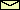# The Pendulum Driven by a Periodic Force

acceleration of gravity = 9.81 m/sec2

For instructions click on here.
For the equation of motion click on here

Suggestions for EXPERIMENTS
1. What happens if the amplitude of driving is set to zero? How does the behavior depend on the length and on the damping? Choose, for example, length = 4 m. Now, compare the behavior for damping less than and greater than 3.13 sec-1.
 Related topics in the lecture room: The harmonic oscillator with damping.
2. Choose length = 1 m, damping = 1 sec-1, and amplitude = 0.2 m (this are the default settings when the applet is started). Now, change the frequency of driving very slowly from 0.2 Hz to 0.8 Hz. Observe the change in the amplitude of oscillation. You can measure it by using the oscilloscope.
 Related topics in the lecture room: Resonance.
3. Choose length = 1 m, damping = 0.4 sec-1, amplitude = 0.2 m and frequency = 0.4 Hz. Depending on the initial condition, the pendulum oscillates either with a large amplitude or with a small one.
 Related topics in the lecture room: Foldover and bistability.
4. Choose length = 1 m, damping = 1.566 sec-1, and frequency = 0.3323 Hz. On the oscilloscope select the angle as the x-axis and the angular velocity as the y-axis. For the scaling of the axes choose 180 and 500, respectively. Now, switch the oscilloscope on and investigate the behavior for the following values of the amplitude: 0.9 m, 1.07 m, 1.15 m, 1.35 m, 1.45 m, 1.47 m, and 1.5 m. You will observe simple periodic orbits, period-doubling bifurcations and deterministic chaos. Note, that with this sequence of the amplitude you will reproduce Fig. 3.4 of in Baker and Gollub's book.
 Related topics in the lecture room: Nonlinear dynamics.

© 1998 Franz-Josef Elmer,Franz-Josef doht Elmer aht unibas doht ch, last modified Friday, June 27, 2011.